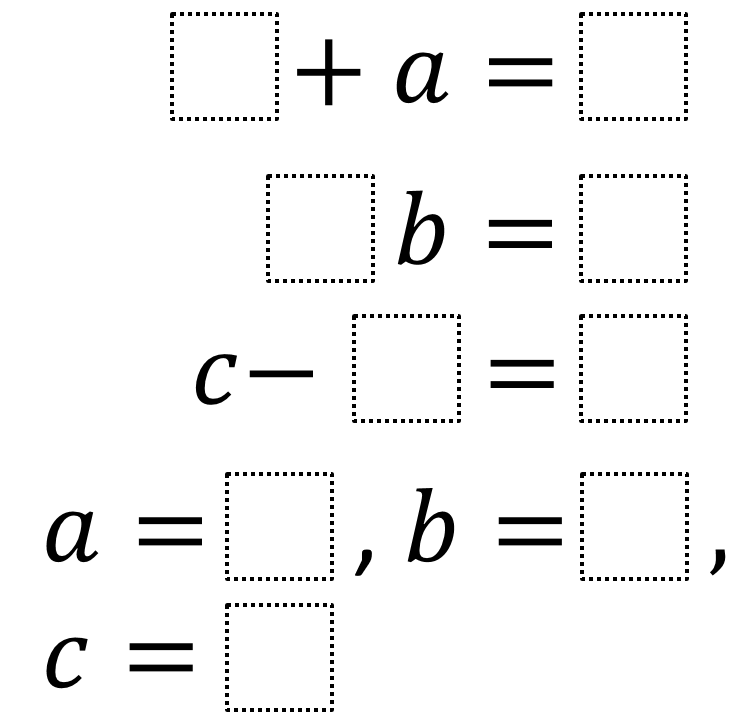# Solving One-Step Equations

Directions: Using the digits 1 to 9 at most one time each, fill in the boxes to make each equation true.### Hint

How does choosing the coefficient or constant affect the variable’s value?
What is the largest possible value that the coefficient of b can be?

One answer from Barb Everhart is:
4 + a = 9
3 * b = 6
c – 7 = 1
a = 5, b = 2, c = 8

Number of Unique Solutions: 16
1: 1 + a = 8, 2b = 6, c – 4 = 5 with a = 7, b = 3, c = 9
2: 1 + a = 8, 2b = 6, c – 5 = 4 with a = 7, b = 3, c = 9
3: 1 + a = 8, 3b = 6, c – 4 = 5 with a = 7, b = 2, c = 9
4: 1 + a = 8, 3b = 6, c – 5 = 4 with a = 7, b = 2, c = 9
5: 4 + a = 9, 2b = 6, c – 1 = 7 with a = 5, b = 3, c = 8
6: 4 + a = 9, 2b = 6, c – 7 = 1 with a = 5, b = 3, c = 8
7: 4 + a = 9, 3b = 6, c – 1 = 7 with a = 5, b = 2, c = 8
8: 4 + a = 9, 3b = 6, c – 7 = 1 with a = 5, b = 2, c = 8
9: 5 + a = 9, 2b = 6, c – 1 = 7 with a = 4, b = 3, c = 8
10: 5 + a = 9, 2b = 6, c – 7 = 1 with a = 4, b = 3, c = 8
11: 5 + a = 9, 3b = 6, c – 1 = 7 with a = 4, b = 2, c = 8
12: 5 + a = 9, 3b = 6, c – 7 = 1 with a = 4, b = 2, c = 8
13: 7 + a = 8, 2b = 6, c – 4 = 5 with a = 1, b = 3, c = 9
14: 7 + a = 8, 2b = 6, c – 5 = 4 with a = 1, b = 3, c = 9
15: 7 + a = 8, 3b = 6, c – 4 = 5 with a = 1, b = 2, c = 9
16: 7 + a = 8, 3b = 6, c – 5 = 4 with a = 1, b = 2, c = 9

Source: Robert Kaplinsky

## Solving One-Step Inequalities with Addition

Directions: Using the digits 0 to 9 at most one time each, place a digit …

1.This answer does not seem to fit the problem (please let me know if I am missing something).

4 + a = 9
3 * b = 6
c – 7 = 1
a = 5, b = 2, c = 8

I am using this problem in PD tomorrow, so please let me know if there is an error in my thinking. 🙂

•Thanks Barb. I’m not sure how that answer got switched into this problem. I can’t remember what answer I originally got. Your’s definitely works though. Probably others too.

2.Another student came up with

1+a=8
3b=6
c-4=5
a=7, b=2, c=9

3.The thought process in this question reminds me of Ken Ken Puzzles. These can be played on-line or you can receive an weekly email with printable puzzles. http://www.kenkenpuzzle.com/teachers/classroom

– I do not work for Ken Ken…just love them.